# Math: Elementary School: Grades 3, 4 and 5 Quiz - Using Symmetry - Properties of 2D Shapes (Questions)

This Math quiz is called 'Using Symmetry - Properties of 2D Shapes' and it has been written by teachers to help you if you are studying the subject at elementary school. Playing educational quizzes is an enjoyable way to learn if you are in the 3rd, 4th or 5th grade - aged 8 to 11.

It costs only \$12.50 per month to play this quiz and over 3,500 others that help you with your school work. You can subscribe on the page at Join Us

This quiz deals with identifying and describing the properties of 2-D shapes, including the number of sides and line symmetry in a vertical line.

In elementary school children are taught to identify some of the properties of 2-dimensional shapes. They will begin to explore this for themselves, perhaps by folding a shape exactly in half - the fold line then shows one line of symmetry.

This quiz will help children to identify the property of symmetry in a variety of 2-dimensional shapes.

Can you recognize the lines of symmetry in these shapes?

1.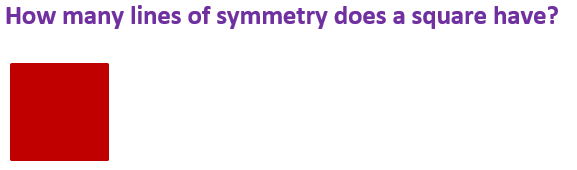[ ] 7 [ ] 4 [ ] 8 [ ] 2
2.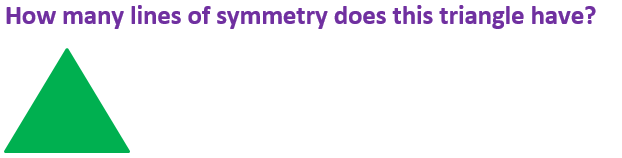[ ] 2 [ ] 6 [ ] 4 [ ] 3
3.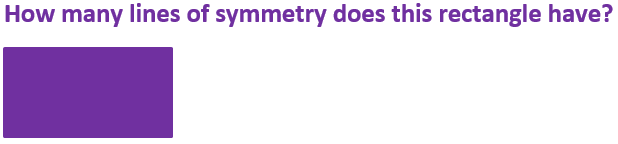[ ] 2 [ ] 4 [ ] 6 [ ] 8
4.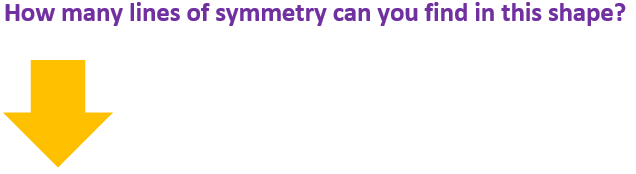[ ] 2 [ ] 1 [ ] 4 [ ] 5
5.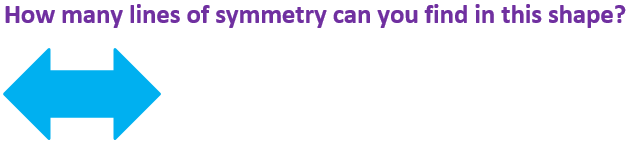[ ] 1 [ ] 3 [ ] 4 [ ] 2
6.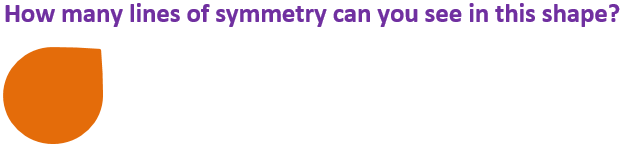[ ] 1 [ ] 2 [ ] 4 [ ] 5
7.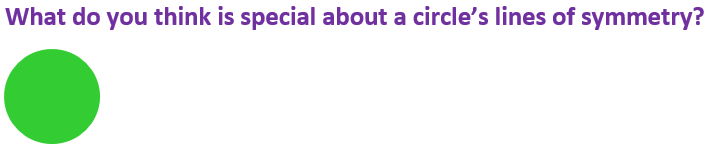[ ] It doesn’t have any [ ] It only has one [ ] It has four [ ] Its lines of symmetry go on for ever
8.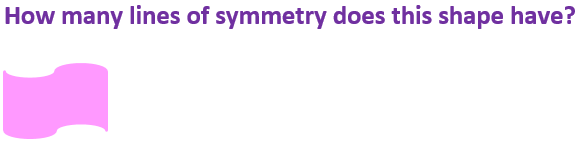[ ] 2 [ ] 4 [ ] 0 [ ] 4
9.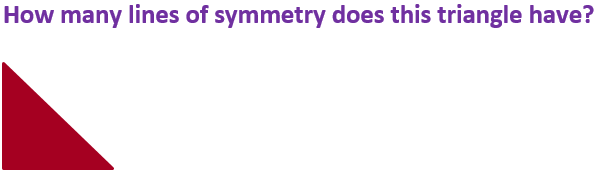[ ] 3 [ ] 9 [ ] 4 [ ] 1
10.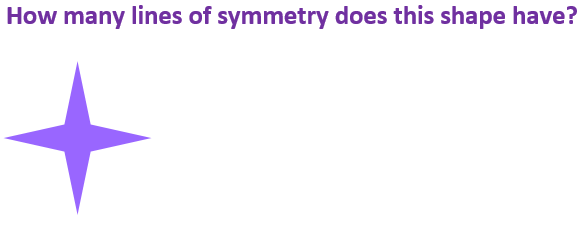[ ] 2 [ ] 6 [ ] 5 [ ] 4Math: Elementary School: Grades 3, 4 and 5 Quiz - Using Symmetry - Properties of 2D Shapes (Answers)
1.[ ] 7 [x] 4 [ ] 8 [ ] 2
From corner to corner, through the middle vertically and horizontally
2.[ ] 2 [ ] 6 [ ] 4 [x] 3
From each point to its opposite straight side
3.[x] 2 [ ] 4 [ ] 6 [ ] 8
This shape doesn’t have lines of symmetry from corner to corner like the square
4.[ ] 2 [x] 1 [ ] 4 [ ] 5
There is only one place this shape could be folded exactly in half – right down the middle
5.[ ] 1 [ ] 3 [ ] 4 [x] 2
The shape could be folded exactly in half from left to right or top to bottom
6.[x] 1 [ ] 2 [ ] 4 [ ] 5
There is only one place where this shape could be folded exactly in half
7.[ ] It doesn’t have any [ ] It only has one [ ] It has four [x] Its lines of symmetry go on for ever
Circles have infinite lines of symmetry – you can fold them exactly in half all the way around!
8.[ ] 2 [ ] 4 [x] 0 [ ] 4
The shape can’t be folded in half exactly at all
9.[ ] 3 [ ] 9 [ ] 4 [x] 1
Only equilateral triangles have three lines of symmetry
10.[ ] 2 [ ] 6 [ ] 5 [x] 4
Try to picture where you could fold the shape exactly in half